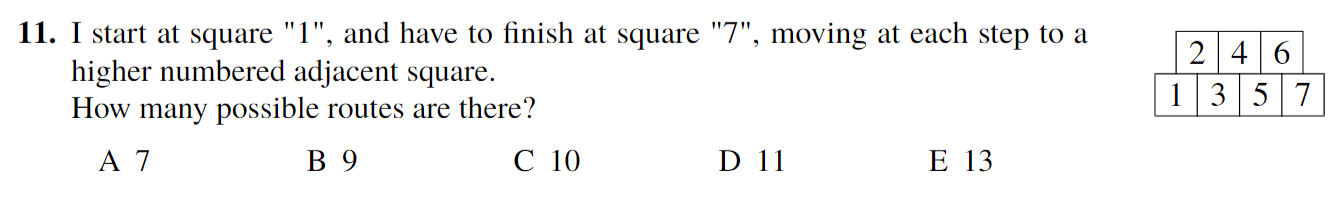# How to solve this grid problem?

• Can anyone tell me how to solve this? And also please can you explain me each step, I get lost pretty quickly. Thank you for your kindness and your help. Really thank you.• @fabulousgrizzly Well, my answer was 13. Essentially, this is path-counting. If you don't know what path-counting is, watch: https://www.youtube.com/watch?v=6neKbdswFXk

So, to get to 2, we have 1 option, 3 we have 2, 4 we have 3 as by path-counting, 5 we have 2+3=5, and 6 we have 5+3=8. Therefore, our answer is 5+8=13.

• The answer should be 13, you find the answer by counting the number of ways to get to each tile, kind of like how you derive the next digits in Pascal's triangle, but you might have not gotten to that yet.

Basically, here's what you do:

There is 1 way for you to get to box 1 (since you start there).

There is 1 way for you to get to box 2 (since you start at 1).

There are 2 ways for you to get to box 3 (one way from 1 and another way from 2).

There are 3 ways for you to get to box 4 (one way from 2 and two ways from 3).

There are 5 ways for you to get to box 5 (two ways from 3 and three ways from 4)

There are 8 ways for you to get to box 6 (three ways from 4 and five ways from 5)

And lastly, there are 13 ways (the answer!!) for you to get to box 7 (five ways from 5 and eight ways from 6)

Reminder that you can't go to a square with a smaller number, which is why box 2 only had 1 possibility, etc.

Hope this helped.

Happy learning,
(Unofficial) The Daily Challenge Team